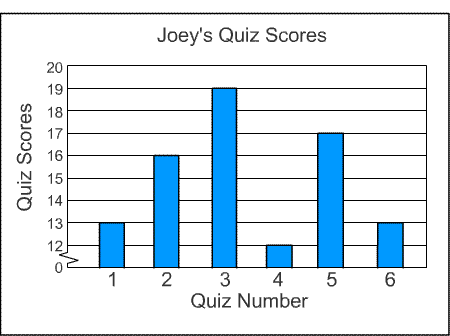Skill 8B
Range, Mode, Median, Mean

Use the data from the bar graph to answer the questions.1. What is the range of the data shown in the bar graph? 2. What is the mode of the data? 3. What is the median of the data? 4. What is the mean of the data?